# Operations with binary numbers

As with decimals we can do operations with binary numbers, whether it be adding, subtracting, multiplying and / or making divisions. Although each of these operations has its rules, they are easy to remember because they are quite simple.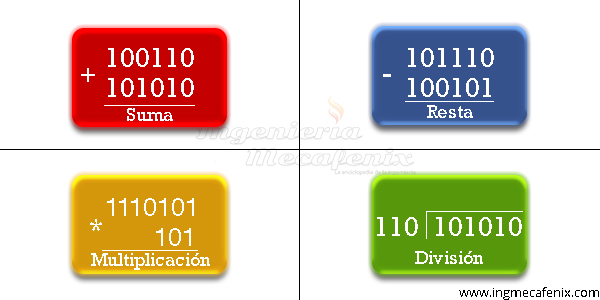### Sum

For the sum of two or more binary numbers we must use 4 fundamental rules that help us and facilitate the work.

Rules

0 + 0 = 0

0 + 1 = 1

1 + 0 = 1

1 + 1 = 0, with carry of 1 in the next row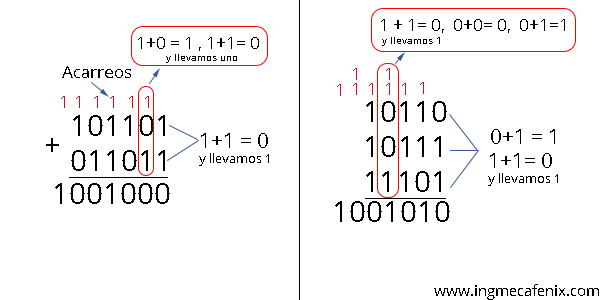### Subtraction

Subtraction is practically the same as the decimal system, since the same term that borrows the number in the next row is used when the number to be subtracted is less than the one that is subtracted.

Rules

0 – 0 = 0

0 – 1 = 1 with carry from 1 to the next row

1 – 0 = 1

1 – 1 = 0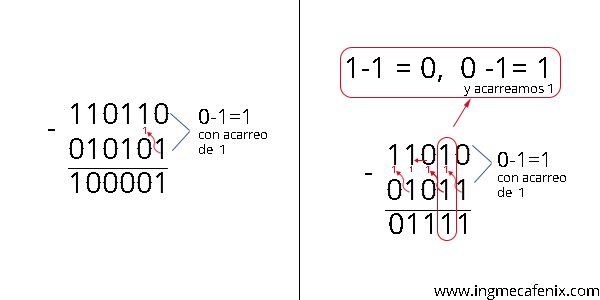### Multiplication

Multiplication is done as in decimal numbers, first multiply and then add the results. Although multiplication is more advanced, in this case it only has two rules. When multiplied by 0 it always gives 0 and when multiplied by 1 it is equal to 1.### Division

This operation is practically the same as divisions with decimal numbers but with a great advantage that only two numbers are used in this system.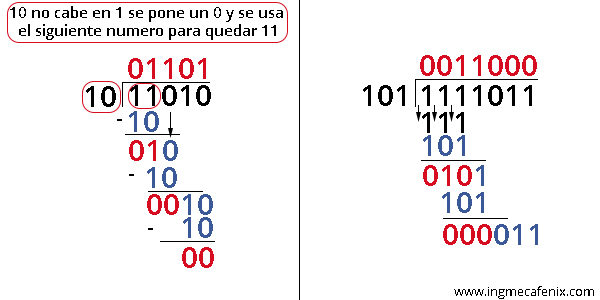## Converting binaries to other systems

### Binary – Decimal

In order to convert from binary to decimal we always have to start with the least significant bit or the number with the least value. Once this bit is located we must start to make a progressive numbering on the binary numbers starting with 2 ^ 0, then 2 ^ 1, 2 ^ 2, 2 ^ 3 and so on until we have all the binary numbers with this new numbering.

As a final step to convert to decimal we have to add all the digits with 1 but with the new numbering. ?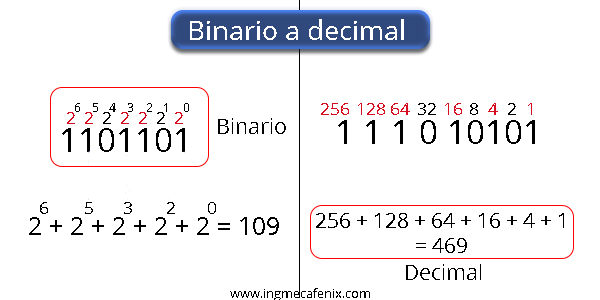### Decimal – binary

To convert from decimal to binary it is quite simple since we take the number and divide it by two, if the result gives an integer it puts 0 and if the result gives a number with decimals it puts 1, the next number is they remove the decimals and divide again, at the end the 1 is always dragged and it is placed on the left side.### Binary – Octal and Octal – Binary

For this conversion, not much knowledge is required and it is quite simple, we only have to decompose the binary number into groups of three, always starting from right to left, if at the end we do not have a complete group, just add the necessary zeros so that it is complete the group.

To convert from octals to binaries the same procedure is done, each number is selected separately and converted into its respective binary.